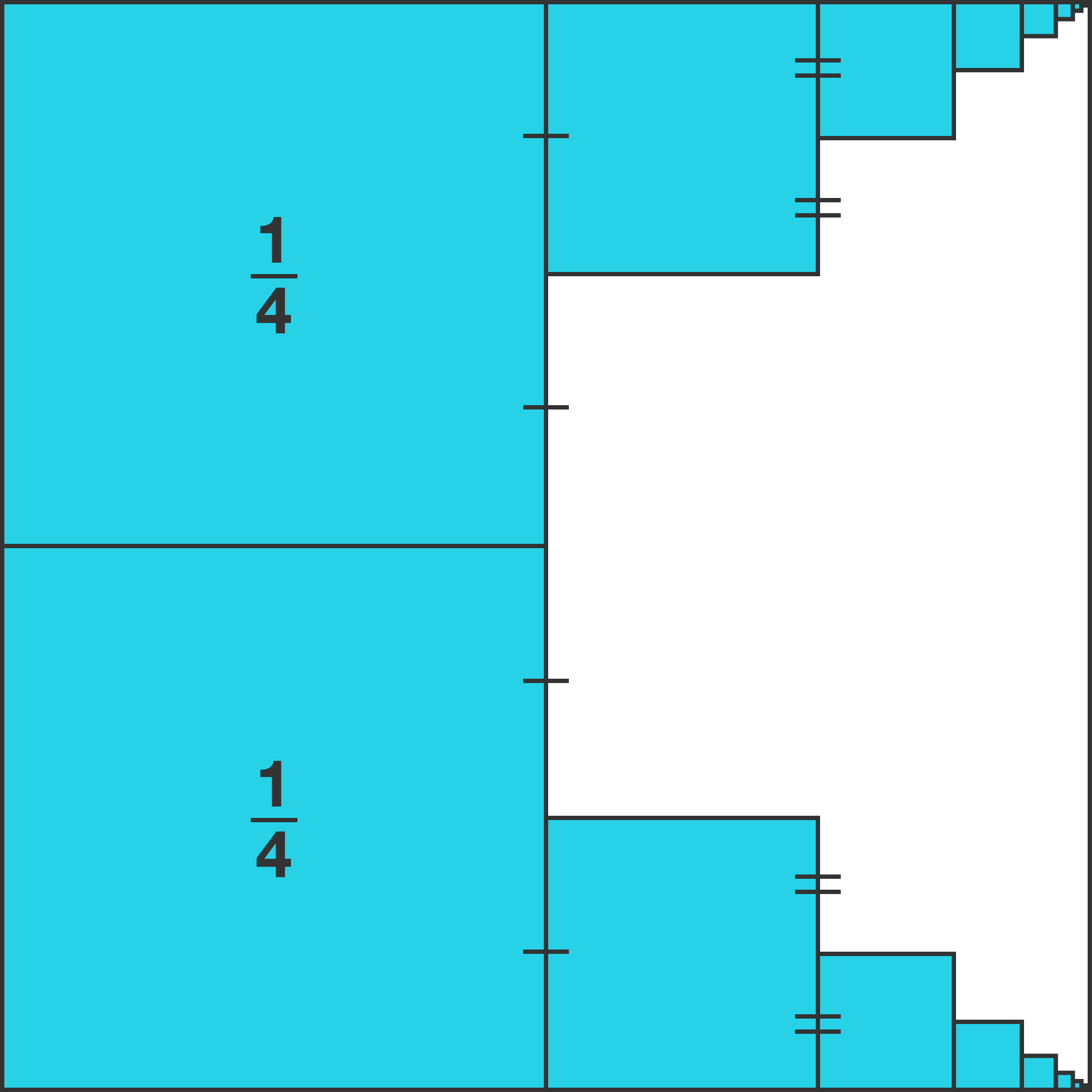# 22 of 100: Squishing Squares

Algebra Level 1The left half of a square is occupied by two smaller blue squares as shown, each a quarter the area of the original large square.

To the right of both squares there is a succession of blue squares, each one connecting to the midpoint of the previous square as shown.

When this process is repeated many times, approximately how much of the original large square is shaded blue?It all adds up more neatly than you might expect. Think about the symmetry of this situation.

×